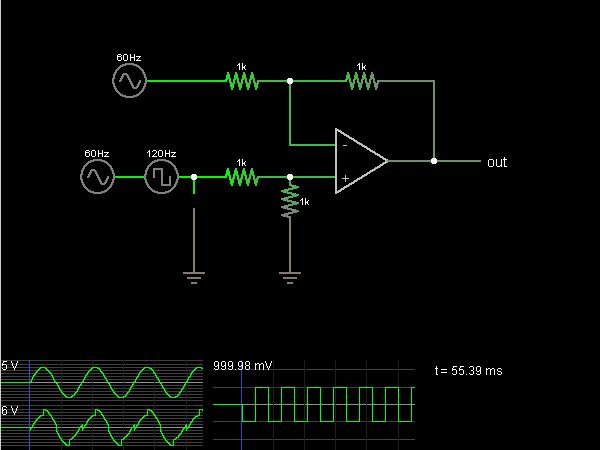# Circuit Simulator - Differential Amplifier (with op-amps)

## Why Differential Amplifier (with op-amps)?

Learn about Differential Amplifier (with op-amps) to improve your skills and design your electronics projects yourself.

## Where can I get Differential Amplifier (with op-amps) Circuit Diagram with Explanation?

IndiaBIX provides you lots of fully solved Differential Amplifier (with op-amps) circuit diagram with detailed explanation and working principles.

## How to design a Differential Amplifier (with op-amps) (electronic circuit)?

You can easily design the Differential Amplifier (with op-amps) circuit by practicing the exercises given below. Here you can design and simulate your own electronic circuits with this Online Circuit Designer and Simulator.### Circuit Description:

This circuit outputs the difference in voltage between two input signals. In this case, the first input is a 60 Hz signal, and the second input is a 60 Hz signal with 120 Hz square wave added in. (In this simulation, the two signals are added simply by connecting the two sources in series, which is convenient but not realistic.)

The second input signal is driving two resistors, which act as a voltage divider, and the + input of the op-amp is connected between them, where the voltage is equal to half the second input signal. The op-amp attempts to keep its – input at the same voltage as the + input. The two resistors on top act as a voltage divider, making the – input halfway between the first input signal and the op-amp output.

If V+ = 1/2 In2 = V = 1/2 (In1 + output), then the output = In2 - In1, or the difference between the two inputs. -- Credits: Mr. Paul Falstad.